Notebook

# Yield Curve as a Hedging Indicator¶

## Objective: Hedge a Traditional 60:40 Portfolio Based on Yield Curve Flatness¶

FRED graph with the current data set being used in this notebook. Click the link below, click download as CSV in the top left corner of the FRED page. Then, on Quantopian, under Notebooks, upload that file in your data folder, and name is 'fredgraph-20.csv'

https://fred.stlouisfed.org/graph/?g=j6BC

To hedge the traditional portfolio, I will present 2 different options:

1. Delevering the entire portfolio and increasing the cash allocation
2. Introducing a hedge asset mix, composed of assets which tend to rise as short rates decrease

For implementing the Yield Curve as a Hedging Indicator, I will calculate a value at which I will either delever or invest moreso in the Hedge Asset Mix. I will take the max value of the calculated Hedge Value from the past 18 months to determine the hedging level used for the current month. A similar methodology was described and demonstrated in a research paper "The Yield Curve as a Leading Indicator: Some Practical Issues" (2006) by Estrella and Turbin. I will assume a return on cash as a constant value of 0.

https://msuweb.montclair.edu/~lebelp/EstrellaYieldCurveIndicatorFRBNY200608.pdf

My Hedge Asset Mix will be composed of: 33% - short equities 33% - long commodity futures (gold, silver, oil) 33% - long bonds

In :
from statsmodels import regression
from quantopian.pipeline import Pipeline
from quantopian.pipeline.data import morningstar
from quantopian.pipeline.factors import CustomFactor
from quantopian.research import run_pipeline
from quantopian.pipeline.classifiers.morningstar import Sector
from quantopian.pipeline.factors import MarketCap
from quantopian.pipeline.data.builtin import USEquityPricing
from quantopian.research.experimental import history
from quantopian.research.experimental import continuous_future
from quantopian.pipeline.factors import SimpleMovingAverage, AverageDollarVolume

import numpy as np
import pandas as pd
import math
import statsmodels.api as sm
import matplotlib.pyplot as plt
import seaborn as sns
import scipy as sc
from odo import odo
from statsmodels.stats.stattools import jarque_bera


## Import Equity and Bond ETF Data¶

In :
# SPY, IWM, QQQ will represent the Equity investments, 60% of the portfolio by cash
# TIP, TLT, IEF, SHY will represent the Bond investments, 40% of the portfolio by cash
start = '2004-04-01'
end = '2010-06-01'
assets = ['SPY','IWM','QQQ','TIP','TLT','IEF','SHY']
data = get_pricing(assets, start_date=start, end_date=end, fields='price')

Out:
Equity(8554 [SPY]) Equity(21519 [IWM]) Equity(19920 [QQQ]) Equity(25801 [TIP]) Equity(23921 [TLT]) Equity(23870 [IEF]) Equity(23911 [SHY])
2004-04-01 00:00:00+00:00 100.647 56.809 35.092 92.439 77.268 77.169 76.451
2004-04-02 00:00:00+00:00 101.472 57.735 35.957 91.067 75.339 75.697 76.183
2004-04-05 00:00:00+00:00 102.252 57.946 36.404 90.571 74.731 75.387 76.110
2004-04-06 00:00:00+00:00 102.030 57.217 36.023 90.693 75.113 75.653 76.202
2004-04-07 00:00:00+00:00 101.507 57.481 35.830 90.154 75.053 75.662 76.220
In :
# Get monthly percent returns
monthly_data = data.resample('M').mean()
mult_returns = monthly_data.pct_change()[1:-1]
mult_returns.columns = assets

Out:
SPY IWM QQQ TIP TLT IEF SHY
2004-05-31 00:00:00+00:00 -0.025206 -0.057881 -0.034218 -0.011532 -0.031118 -0.022325 -0.005131
2004-06-30 00:00:00+00:00 0.028766 0.038413 0.042000 0.005046 0.006407 0.002386 -0.001319
2004-07-31 00:00:00+00:00 -0.022961 -0.027454 -0.041469 0.014896 0.030382 0.020445 0.005310
2004-08-31 00:00:00+00:00 -0.013650 -0.036608 -0.043498 0.017106 0.025490 0.018528 0.005013
2004-09-30 00:00:00+00:00 0.028000 0.055970 0.036585 0.007670 0.025874 0.014574 0.002002

## Import Yield Curve Data from FRED¶

1. 5 Year Treasury Rate - 3-Month Treasury Bill Rate
In :
# Imports economic data as a pandas Data Frame
econ_data = local_csv('fredgraph-20.csv')
econ_data.columns = [var_names]

Out:
0 2004-06-01 2.48
1 2004-07-01 2.26
2 2004-08-01 1.74
3 2004-09-01 1.67
4 2004-10-01 1.39
In :
# Merge Economic and Bond ETF data into one Data Frame
all_variable_names = var_names+assets
x=0
temp=[]
for y in econ_data.iloc[x]:
temp.append(y)
for y in mult_returns.iloc[x]:
temp.append(y)
temp_dfs = pd.DataFrame([temp],columns=all_variable_names)
all_data = pd.DataFrame(temp_dfs)

In :
for x in range(1,73):
temp=[]
for y in econ_data.iloc[x]:
temp.append(y)
for y in mult_returns.iloc[x]:
temp.append(y)
temp_dfs = pd.DataFrame([temp],columns=all_variable_names)
all_data = all_data.append(temp_dfs)

In :
all_data.index = range(73)

In :
all_data.head(2)

Out:
Date Yield_Curve_Spread SPY IWM QQQ TIP TLT IEF SHY
0 2004-06-01 2.48 -0.025206 -0.057881 -0.034218 -0.011532 -0.031118 -0.022325 -0.005131
1 2004-07-01 2.26 0.028766 0.038413 0.042000 0.005046 0.006407 0.002386 -0.001319
In :
all_data.tail(2)

Out:
Date Yield_Curve_Spread SPY IWM QQQ TIP TLT IEF SHY
71 2010-05-01 1.94 0.040523 0.064944 0.047434 0.008501 -0.002417 -0.003106 -0.000388
72 2010-06-01 1.61 -0.058834 -0.050788 -0.058388 0.017577 0.068059 0.032524 0.005750
In :
all_data.corr('spearman')

Out:
Yield_Curve_Spread SPY IWM QQQ TIP TLT IEF SHY
Yield_Curve_Spread 1.000000 0.017773 0.071045 0.071631 0.135179 -0.039557 -0.018513 0.028264
SPY 0.017773 1.000000 0.902104 0.907257 0.000617 -0.185734 -0.257713 -0.350025
IWM 0.071045 0.902104 1.000000 0.846076 -0.078459 -0.200605 -0.280143 -0.356565
QQQ 0.071631 0.907257 0.846076 1.000000 -0.017617 -0.229637 -0.279279 -0.382173
TIP 0.135179 0.000617 -0.078459 -0.017617 1.000000 0.553067 0.632050 0.549642
TLT -0.039557 -0.185734 -0.200605 -0.229637 0.553067 1.000000 0.908120 0.708410
IEF -0.018513 -0.257713 -0.280143 -0.279279 0.632050 0.908120 1.000000 0.825558
SHY 0.028264 -0.350025 -0.356565 -0.382173 0.549642 0.708410 0.825558 1.000000
1. Define a function, with Yield Curve data as the input, and a hedging level as an output
2. Calculate the 60/40 Portfolio Returns In-Sample
3. Calculate the Hedged Portfolios Returns In-Sample
4. Compare the returns of the unhedged portfolio to that of the hedged portfolio

## $L(x)=\frac{N}{1+e^{-k(x-x_0)}}$¶

where N=1, k=15, $x_0$=0.1, we have:

## $L(x)=\frac{1}{1+e^{-15(x-0.1)}}$¶

In :
x = np.linspace(-2,3,101)
y = []
e = math.exp(1)
for i in x:
y.append(1/(1+(e**(15*(i-0.1)))))
plt.plot(x,y)
plt.ylim(-0.2,1.2)
plt.ylabel('Hedging Value',fontsize=12)
plt.title('Hedging Function',fontsize=16);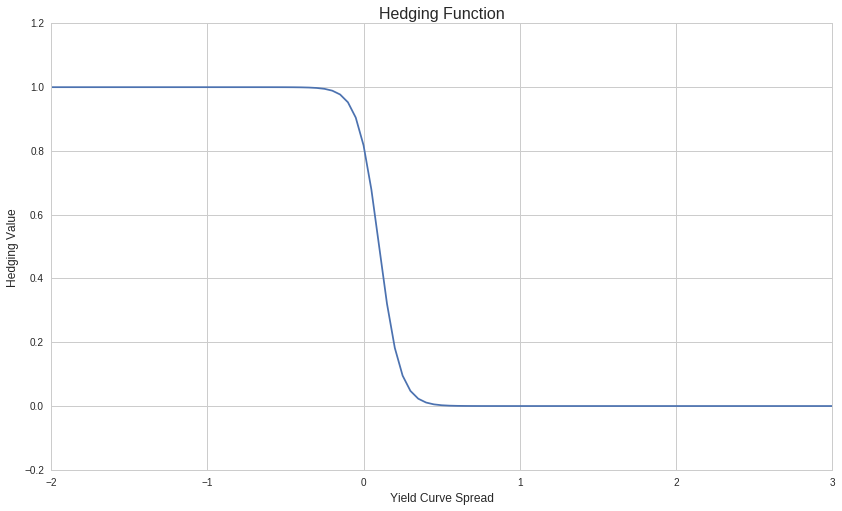In :
x = np.linspace(0,72,72)
sinx = []
y = []
e = math.exp(1)
for i in x:
noise = np.random.normal(0,0.2)
sinx.append((math.sin((i/10)-4.5)+1)+noise)
y.append(1/(1+(e**(15*(i-0.1)))))
plt.plot(x,sinx)
plt.legend(['Sin(x) with Random, Normal Noise','Real Yield Curve Data'])
plt.ylim(-1,3)
plt.xlim(0,72)
plt.xlabel('Time',fontsize=12);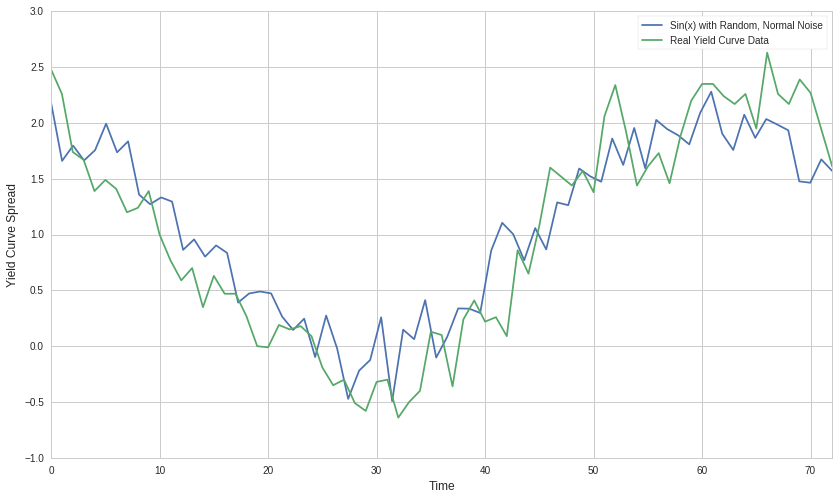In :
x = np.linspace(0,288,288)
sinx = []
theo_hedge_val = []
e = math.exp(1)
for i in x:
noise = np.random.normal(0,0.2)
sinx.append((math.sin((i/10)-4.5)+1)+noise)
theo_hedge_val.append(1/(1+(e**(15*(sinx[-1]-0.1)))))
plt.plot(x,theo_hedge_val)
plt.legend(['Theoretical Hedge Level'])
plt.ylim(-1,3)
plt.xlim(0,288)
plt.ylabel('Hedging Level',fontsize=12)
plt.xlabel('Theoretical Time Assuming Yield Curve Spread follows Sin(x) Model',fontsize=12);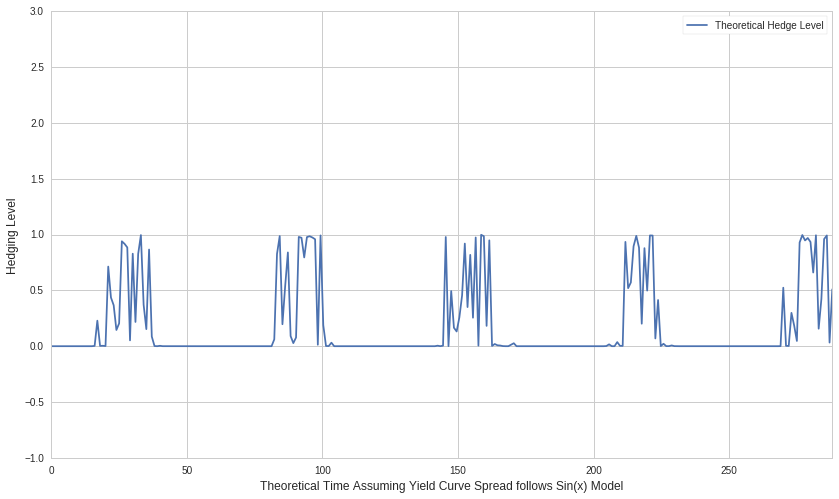### 2. Calculate the 60/40 Portfolio Returns In-Sample¶

I am evenly allocating funds to ETF's within the Equity and Bond portions based on Initial Cash Value

In :
equity_portion = (all_data.SPY + all_data.IWM + all_data.QQQ) / 3
bond_portion = (all_data.TIP + all_data.TLT + all_data.IEF + all_data.SHY) / 4
traditional_portfolio_returns = (0.60 * equity_portion) + (0.40 * bond_portion)
# Shift forward the portfolio returns by 12 months to match the 12-month forward method for hedging
plt.xlim(12,72)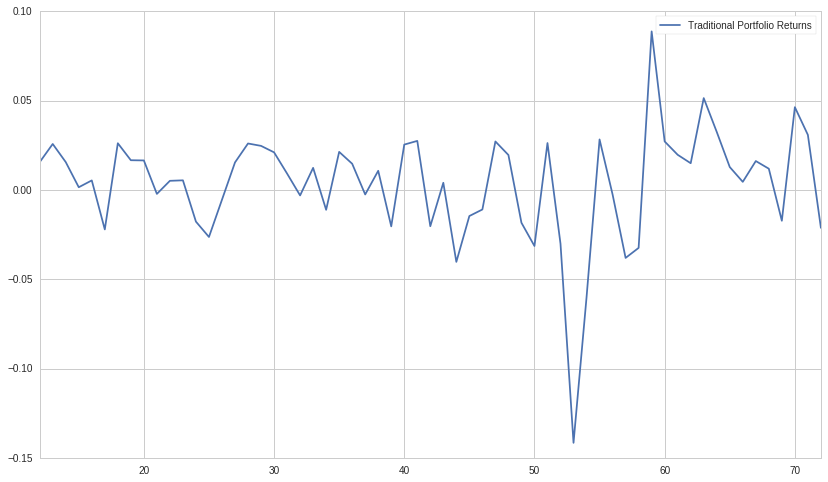In :
traditional_sharpe = traditional_portfolio_returns.mean()/traditional_portfolio_returns.std()
print("Sharpe Ratio for 60/40 Portfolio: " + str(traditional_sharpe))

Sharpe Ratio for 60/40 Portfolio: 0.112165902059


### 3. Calculate the Hedged Portfolios Returns In-Sample¶

In :
hedged_portfolio_1 = []
hedge_value = []
hedge_level_max = []
hedge_value.append(1/(1+(e**(15*(temp-0.1)))))
for i in range(18):
hedge_level_max.append(0)
for i in range(18,len(hedge_value)):
hedge_level_max.append(max(hedge_value[i-17:i+1]))

In :
plt.plot(hedge_value)
plt.plot(hedge_level_max)
plt.legend(['Hedge Value', '12-Month Max Hedge Value'])
plt.ylim(0,1.2)
plt.xlim(0,72)
plt.xlabel('Real Time')
plt.ylabel('Hedge Level');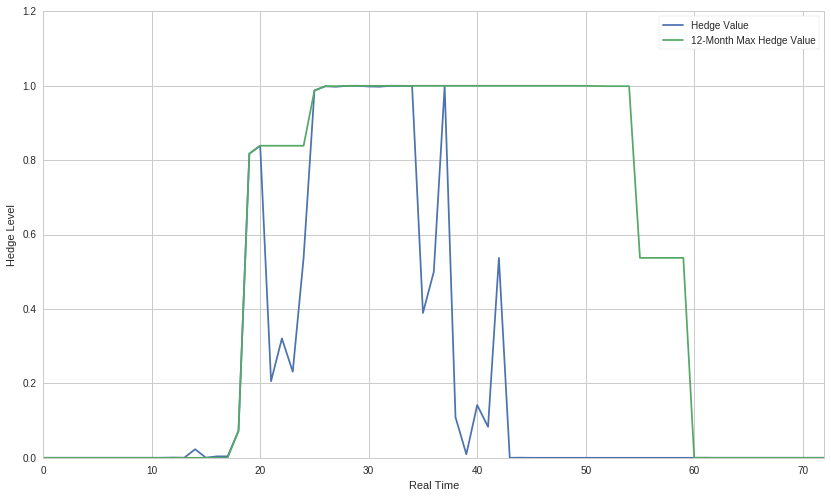In :
hedged_portfolio_1 = []
for i in range(len(hedge_level_max)):
cash_level = hedge_level_max[i]
eq_level = (1-cash_level) * 0.60
bnd_level = (1-cash_level) * 0.40
hedged_portfolio_1.append((0*cash_level)+(eq_level*equity_portion[i])+(bnd_level*bond_portion[i]))


Import Futures Data for Hedge Asset Mix

In :
gc = continuous_future('GC', offset=0, roll='volume', adjustment='mul')
si = continuous_future('SV', offset=0, roll='volume', adjustment='mul')
cl = continuous_future('CL', offset=0, roll='volume', adjustment='mul')
#vx = continuous_future('VX', offset=0, roll='volume', adjustment='mul')

futures_active = history(
[gc,si,cl],
fields='price',
frequency='daily',
start=start,
end=end
)


Out:
ContinuousFuture(92115984680288256 [GC, 0, volume, mul]) ContinuousFuture(95514575121743872 [SV, 0, volume, mul]) ContinuousFuture(90999980378095616 [CL, 0, volume, mul])
2004-04-01 00:00:00+00:00 527.0 10.146 100.19
2004-04-02 00:00:00+00:00 521.8 10.195 99.78
In :
futures_names = ['GC','SV','CL']
monthly_futures_data = futures_active.resample('M').mean()
futures_mult_returns = monthly_futures_data.pct_change()[1:-1]
futures_mult_returns.columns = futures_names

Out:
GC SV CL
2004-05-31 00:00:00+00:00 -0.049195 -0.169631 0.110628
2004-06-30 00:00:00+00:00 0.017399 -0.005255 -0.054567
2004-07-31 00:00:00+00:00 0.015579 0.084189 0.060244
2004-08-31 00:00:00+00:00 0.006034 0.050856 0.108639
2004-09-30 00:00:00+00:00 0.009794 -0.042591 0.035459
In :
commodity_portion = (futures_mult_returns.GC+futures_mult_returns.SV+futures_mult_returns.CL) / 3
hedged_portfolio_2 = []
HAM_returns = []
for i in range(len(hedge_level_max)):
HAM = ((equity_portion[i]*-0.33)+(commodity_portion[i]*0.33)+(bond_portion[i]*0.33))
HAM_returns.append(HAM)
hedge_mix_level = 0.5*hedge_level_max[i]
eq_level = (1-hedge_mix_level) * 0.60
bnd_level = (1-cash_level) * 0.40
hedged_portfolio_2.append((hedge_mix_level*HAM)+(eq_level*equity_portion[i])+(bnd_level*bond_portion[i]))


### 4. Compare the returns of the unhedged portfolio to that of the hedged portfolio¶

In :
plt.plot(traditional_portfolio_returns,':',linewidth=5)
plt.plot(hedged_portfolio_1,'-.',linewidth=8)
plt.plot(hedged_portfolio_2,'--',linewidth=3)
plt.xlim(18,72)
plt.ylabel('Returns')
plt.xlabel('Real Time')
plt.legend(['Traditional Portfolio','Cash-Hedging Portfolio','Hedge Asset Mix Portfolio'])
plt.title('Distribution of Returns');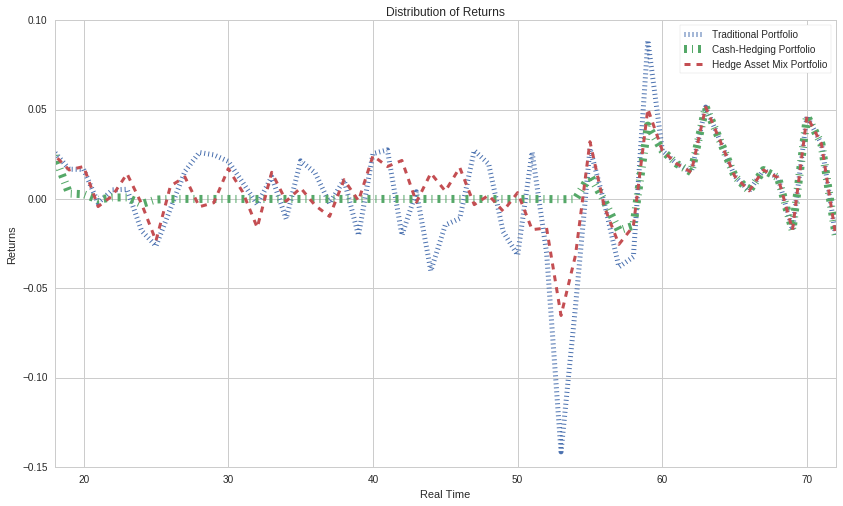In :
hedge1_sharpe = np.mean(hedged_portfolio_1) / np.std(hedged_portfolio_1)
hedge2_sharpe = np.mean(hedged_portfolio_2) / np.std(hedged_portfolio_2)

In :
print("Sharpe Ratio for 60/40 Portfolio: " + str(traditional_sharpe))
print("Sharpe Ratio for Hedged Portfolio 1: " + str(hedge1_sharpe))
print("Sharpe Ratio for Hedged Portfolio 2: " + str(hedge2_sharpe))

Sharpe Ratio for 60/40 Portfolio: 0.112165902059
Sharpe Ratio for Hedged Portfolio 1: 0.313356373261
Sharpe Ratio for Hedged Portfolio 2: 0.262931469197


Given that the Sharpe Ratios for both the Hedged Portfolios are greater than the Sharpe Ratio of the Traditional 60/40 Portfolio, the Yield Curve may be a valuable predictor for hedging portfolios. To recap, we calculated a degree to which we should hedge our portfolio based off the spread of the yield curve in the past 18 months. Using a logistic model, we hedged greater as the spread decreased, especially for values less then to 0.1. In conclusion, this methodology proved to be an effective way of reducing the volatility of our portfolio's returns in relation to the mean return of the portfolio. Investors may be able to achieve higher sharpe ratios by deploying such hedging techniques using other macroeconomic factors as indicators as well.